# Cesium中的图形技术：Primitive API 高级

Geometry and Appearances · CesiumGS/cesium Wiki (github.com)

# 创建自定义几何图形和外观

Cesium支持许多常见的图形，开箱即用。但是，有时候这些预置的图形不一定能满足需求。

# 几何图形

Cesium 中的 Geometry 支持索引三角形、未索引三角形、线框渲染和点渲染。开始，我们先为四面体（三棱锥）创建它的几何形状，即四个等边三角形。首先，在 Source/Core/ 下创建一个 TetrahedronGeometry.js

import Cartesian3 from './Cartesian3'
import ComponentDatatype from './ComponentDatatype'
import PrimitiveType from './PrimitiveType'
import BoundingSphere from './BoundingSphere'
import GeometryAttribute from './GeometryAttribute'
import GeometryAttributes from './GeometryAttributes'
import GeometryPipeline from './GeometryPipeline'
import VertexFormat from './VertexFormat'
import Geometry from './Geometry'

function TetrahedronGeometry() {
const negativeRootTwoOverThree = -Math.sqrt(2.0) / 3.0;
const negativeOneThird = -1.0 / 3.0;
const rootSixOverThree = Math.sqrt(6.0) / 3.0;
const positions = new Float64Array(4 * 3);

// 四面体有4个三角形，共计12个点，但是由于重合的关系，可以只记录4个点
// 点0 坐标
positions = 0.0;
positions = 0.0;
positions = 1.0;

// 点1 坐标
positions = 0.0;
positions = (2.0 * Math.sqrt(2.0)) / 3.0;
positions = negativeOneThird;

// 点2 坐标
positions = -rootSixOverThree;
positions = negativeRootTwoOverThree;
positions = negativeOneThird;

// 点3 坐标
positions = rootSixOverThree;
positions = negativeRootTwoOverThree;
positions = negativeOneThird;

// 创建顶点属性中的坐标
const attributes = new GeometryAttributes({
position : new GeometryAttribute({
componentDatatype : ComponentDatatype.DOUBLE,
componentsPerAttribute : 3,
values : positions
})
});

const indices = new Uint16Array(4 * 3);

// 后面的三角形用到 0、1、2 号点坐标
indices = 0;
indices = 1;
indices = 2;

// 左边的三角形用到 0、2、3 号点坐标
indices = 0;
indices = 2;
indices = 3;

// 右边的三角形用到 0、3、1 号点坐标
indices = 0;
indices = 3;
indices = 1;

// 下面的三角形用到 2、1、3 号点坐标
indices = 2;
indices = 1;
indices = 3;

// 指定此四面体的各种属性
this.attributes = attributes;
this.indices = indices;
this.primitiveType = PrimitiveType.TRIANGLES;
this.boundingSphere = undefined;
}

export default TetrahedronGeometry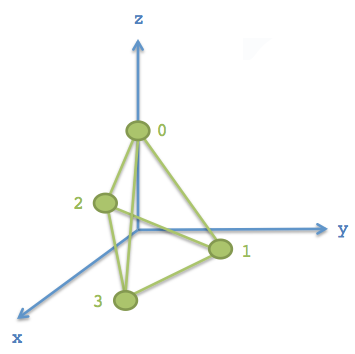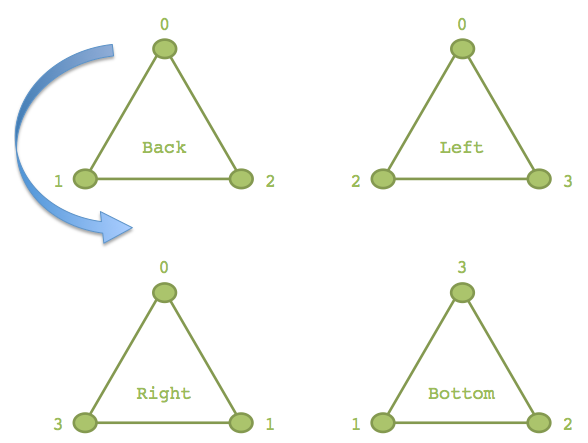“Back” 三角形这儿有个蓝箭头，表示的是顶点的顺序，现在是逆时针。使用右手定则，可以判断它的这个三角形的“外面”是朝向屏幕的。Cesium 使用逆时针顺序来定义三角形的面朝向。

this.attributes = attributes;
this.indices = indices; // 可选
this.primitiveType = Cesium.PrimitiveType.TRIANGLES;
this.boundingSphere = undefined; // 可选

• attributes - 一个 GeometryAttributes 对象，每个顶点属性都存储在这个对象中，顶点属性包括但不限于：坐标、法线、颜色值、uv等
• indices - 索引数组，使用索引可以减少坐标个数，节约内存
• primitiveType - 几何图元的类型，这里使用了 Cesium.PrimitiveType.TRIANGLES，即每三个顶点被解析为一个三角形渲染。
• boundingSphere - 可选参数，包围整个几何形状的球体，可以辅助剔除来提高性能。

## ① 包围球

this.boundingSphere = BoundingSphere.fromVertices(positions);


BoundingSphere 有一个 fromVertices() 函数来计算紧密包围几何形状所有坐标的包围球。不过，通常在其他情况下，可以用简单的几何知识来更快创建它。由于预先知道这个四面体的顶点坐标位于半径为1的球体上，所以可以用半径为1的球面作为边界球：

this.boundingSphere = new BoundingSphere(new Cartesian3(0.0, 0.0, 0.0), 1.0);


## ② 使用 Primitive API 绘制一个四面体

const widget = new Cesium.CesiumWidget('cesiumContainer');
const scene = widget.scene;
const ellipsoid = widget.centralBody.ellipsoid;

// 看似复杂，其实只是对经纬度 (-100, 40) 这个点做垂直地表向上平移200km的计算，并将几何体放大50w倍（即变成500km那么大），返回矩阵而已
const modelMatrix = Cesium.Matrix4.multiplyByUniformScale(
Cesium.Matrix4.multiplyByTranslation(
Cesium.Transforms.eastNorthUpToFixedFrame(ellipsoid.cartographicToCartesian(
Cesium.Cartographic.fromDegrees(-100.0, 40.0))), // e-n-u计算，返回局部到世界坐标的转换矩阵
new Cesium.Cartesian3(0.0, 0.0, 200000.0)), // 平移计算，矩阵·平移向量
500000.0); // 缩放计算，矩阵·50w

const instance = new Cesium.GeometryInstance({
geometry : new Cesium.TetrahedronGeometry(), // 如果直接写在代码而不是构建出来的，可以直接 new TetrahedronGeometry()
modelMatrix : modelMatrix,
attributes : {
color : Cesium.ColorGeometryInstanceAttribute.fromColor(Cesium.Color.WHITE) // 快捷计算顶点颜色
}
});

/* 使用 Primitive API 绘制几何 */
geometryInstances : instance,
appearance : new Cesium.PerInstanceColorAppearance({
flat : true,
translucent : false
})
}));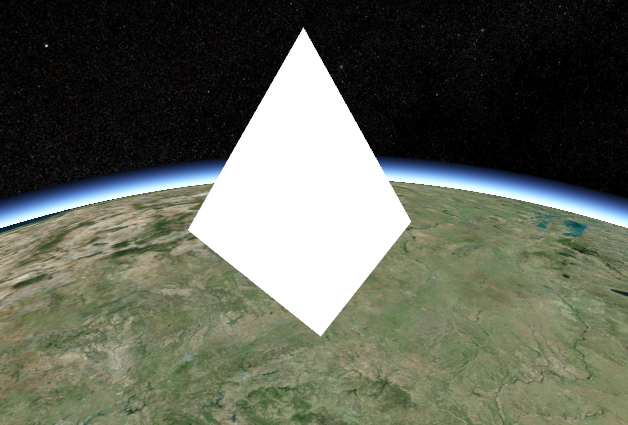GeometryPipeline.toWireframe 方法将几何图形转换为 primitiveType 为 LINE 的模式。

const instance = new Cesium.GeometryInstance({
geometry : Cesium.GeometryPipeline.toWireframe(new Cesium.TetrahedronGeometry()),
modelMatrix : modelMatrix,
attributes : {
color : Cesium.ColorGeometryInstanceAttribute.fromColor(Cesium.Color.WHITE)
}
});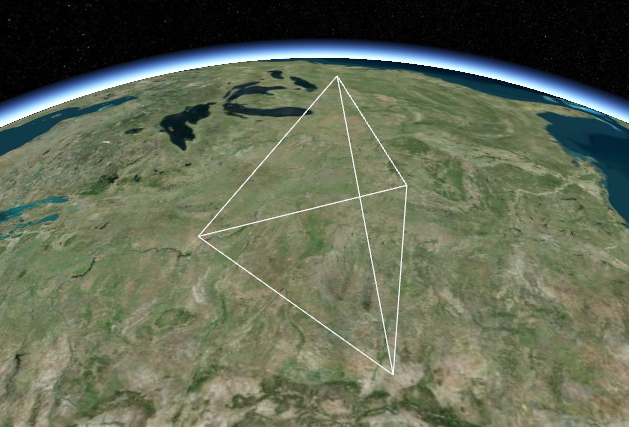## ③ 添加法线出现阴影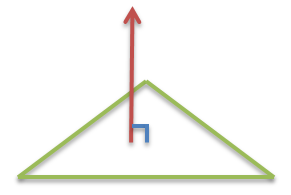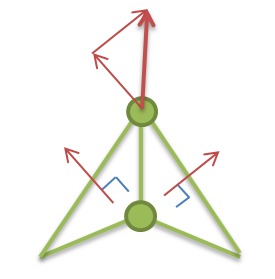// ... 上面省略
const boundingSphere = new BoundingSphere(new Cartesian3(0.0, 0.0, 0.0), 1.0);

// 主要是改这里
const geometry = GeometryPipeline.computeNormal(new Geometry({
attributes: attributes,
indices: indices,
primitiveType: PrimitiveType.TRIANGLES,
boundingSphere: boundingSphere
}));

this.attributes = geometry.attributes;
this.indices = geometry.indices;
this.primitiveType = geometry.primitiveType;
this.boundingSphere = geometry.boundingSphere;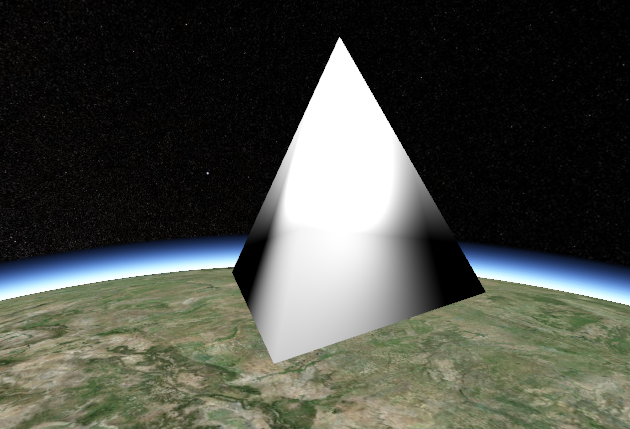scene.primitives.add(Cesium.createTangentSpaceDebugPrimitive({
geometry: tetrahedron,
modelMatrix: modelMatrix,
length: 0.2
}));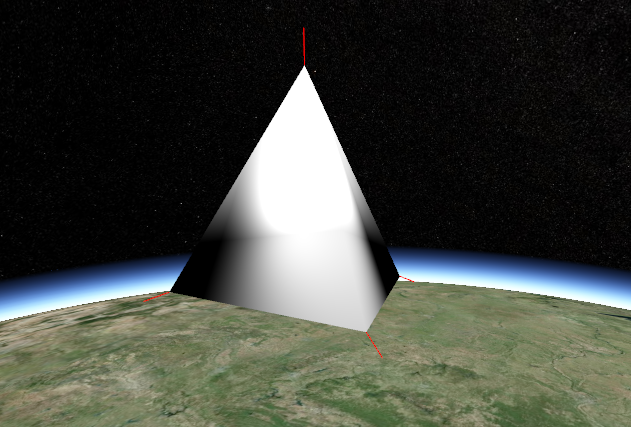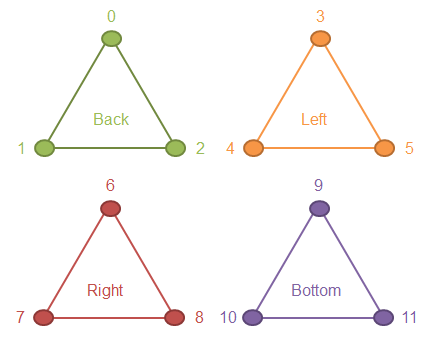var positions = new Float64Array(4 * 3 * 3); // 4个三角形，每个有3个坐标，一个坐标有3个float分量
// back triangle
positions = 0.0;
positions = 0.0;
positions = 1.0;
positions = 0.0;
positions = (2.0 * Math.sqrt(2.0)) / 3.0;
positions = negativeOneThird;
positions = -rootSixOverThree;
positions = negativeRootTwoOverThree;
positions = negativeOneThird;

// left triangle
positions = 0.0;
positions = 0.0;
positions = 1.0;
positions = -rootSixOverThree;
positions = negativeRootTwoOverThree;
positions = negativeOneThird;
positions = rootSixOverThree;
positions = negativeRootTwoOverThree;
positions = negativeOneThird;

// right triangle
positions = 0.0;
positions = 0.0;
positions = 1.0;
positions = rootSixOverThree;
positions = negativeRootTwoOverThree;
positions = negativeOneThird;
positions = 0.0;
positions = (2.0 * Math.sqrt(2.0)) / 3.0;
positions = negativeOneThird;

// bottom triangle
positions = -rootSixOverThree;
positions = negativeRootTwoOverThree;
positions = negativeOneThird;
positions = 0.0;
positions = (2.0 * Math.sqrt(2.0)) / 3.0;
positions = negativeOneThird;
positions = rootSixOverThree;
positions = negativeRootTwoOverThree;
positions = negativeOneThird;

var indices = new Uint16Array(4 * 3); // 12个顶点索引，各自独立

// back triangle
indices = 0;
indices = 1;
indices = 2;

// left triangle
indices = 3;
indices = 4;
indices = 5;

// right triangle
indices = 6;
indices = 7;
indices = 8;

// bottom triangle
indices = 9;
indices = 10;
indices = 11;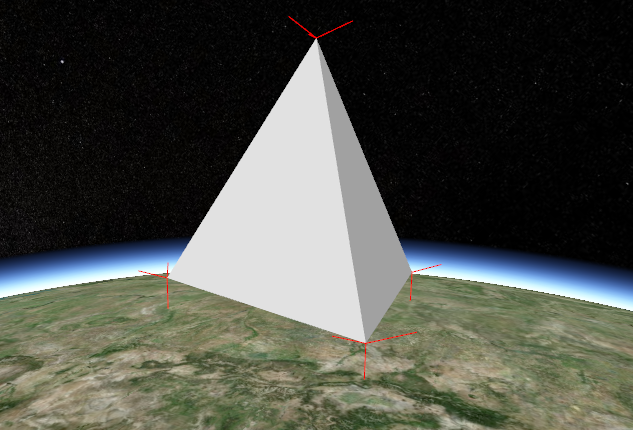## ④ 使用 WebWorker

import TetrahedronGeometry from '../Core/TetrahedronGeometry' // 这个看你喜好咯
import PrimitivePipeline from '../Scene/PrimitivePipeline'

function createTetrahedronGeometry(parameters, transferableObjects) {
const geometry = TetrahedronGeometry.createGeometry();
PrimitivePipeline.transferGeometry(geometry, transferableObjects);

return {
geometry : geometry,
index : parameters.index
};
}


TetrahedronGeometry.createGeometry = function() {
return new Geometry({
attributes : attributes,
indices : indices,
primitiveType : PrimitiveType.TRIANGLES,
boundingSphere : new BoundingSphere(new Cartesian3(0.0, 0.0, 0.0), 1.0)
});
};


this._workerName = 'createTetrahedronGeometry';
// this 指的是 TetrahedronGeometry


posted @ 2020-11-30 01:21  四季留歌  阅读(2593)  评论(0编辑  收藏  举报